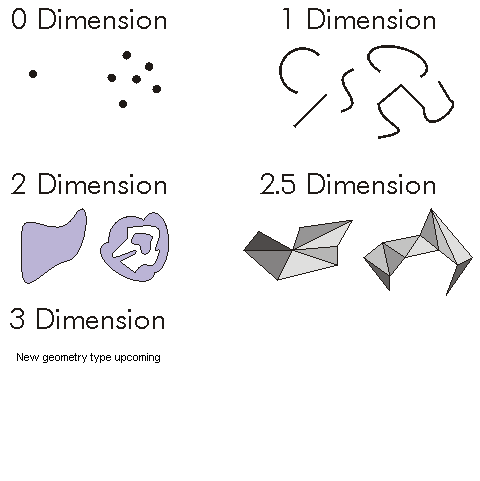This document is archived and information here might be outdated.  Recommended version.

esriGeometryDimension Constants (ArcObjects .NET 10.8 SDK)
 ArcObjects Help for .NET developers > ArcObjects Help for .NET developers > ArcObjects namespaces > Geometry > ESRI.ArcGIS.Geometry > Constants > E > esriGeometryDimension Constants
 ArcGIS Developer Help

# esriGeometryDimension Constants

The topological dimension of a geometry.

Constant Value Description
esriGeometry0Dimension 1 A zero dimensional geometry (such as a point or multipoint).
esriGeometry1Dimension 2 A one dimensional geometry (such as a polyline).
esriGeometry2Dimension 4 A two dimensional geometry (such as a polygon).
esriGeometry25Dimension 5 A 2.5D geometry (such as a surface mesh).
esriGeometry3Dimension 6 A 3D geometry.
esriGeometryNoDimension -1 The dimension is unknown or unspecified.

#### Product Availability

Available with ArcGIS Engine, ArcGIS Desktop, and ArcGIS Server.

#### Remarks

Describes the dimensionality of the geometry object. Each dimension corresponds to a direction of orthogonal movement. Therefore, Zero Dimensions describes a point with no extent beyond the point itself. One Dimension describes a line where the dimension of movement extends along the length of the line. Two Dimensions describes a surface where the movement can occur along two parametric orthogonal axes to create an Area. Three Dimensions describes a space with three parametric directions of movement (height, width, and depth). 2.5 Dimensions is a pseudo-dimensional construct that refers to a functional surface, represented by a planar Two Dimensional object with Z attributes. A No Dimensional object (not to be confused with a Zero Dimensional point) is an object for which dimensionality is not properly defined.

````esriGeometry0Dimension   = Points and MultipointsesriGeometry1Dimension   = Segments, Paths, and PolylinesesriGeometry2Dimension   = Envelopes, Rings, Polygons and MultipatchesesriGeometry25Dimension  = ZAware esriGeometry2Dimension objectsesriGeometry3Dimension   = New upcoming geometry typeesriGeometryNoDimension  = GeometryBags    `
```

``

ViperPin tool